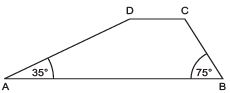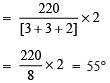Courses

# Very Short Answer Type Questions- Quadrilaterals Class 9 Notes | EduRev

## Class 9 Mathematics by Full Circle

Created by: Full Circle

## Class 9 : Very Short Answer Type Questions- Quadrilaterals Class 9 Notes | EduRev

The document Very Short Answer Type Questions- Quadrilaterals Class 9 Notes | EduRev is a part of the Class 9 Course Class 9 Mathematics by Full Circle.
All you need of Class 9 at this link: Class 9

Question 1. In the adjoining figure, ABCD is a trapezium in which AB || DC. If ∠A = 35º and ∠B = 75º, then find ∠C and ∠D.
Solution:
AB || DC and AD is a transversal.
∴ ∠A + ∠D = 180º
⇒ ∠D = 180° - ∠A
= 180° - 35° = 145°Similarly, ∠B + ∠C = 180º
⇒ ∠C = 180° - 75° = 105°
Thus, ∠C = 105°∠D = 145°

Question 2. Fill in the blanks:
(i) In a parallelogram, opposite angles are ____.(ii) A ____ of a parallelogram divides it into two congruent triangles. (iii) The sum of the angles of a quadrilateral is ____. (iv ) In a parallelogram, the opposite sides are parallel and _____.
Solution:
(i) equal
(ii) diagonal
(iii) 360°
(iv) equal

Question 3. One angle of a quadrilateral is 140° and other three angles are in the ratio of 3 : 3 : 2.
Find the measure of the smallest angle of the quadrilateral.
Solution:
Remaining three angles = 360° - 140° = 220°
Ratio is 3 : 3 : 2
∴ The smallest angle132 docs

,

,

,

,

,

,

,

,

,

,

,

,

,

,

,

,

,

,

,

,

,

;